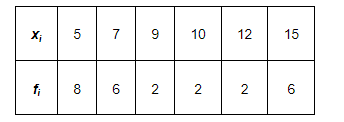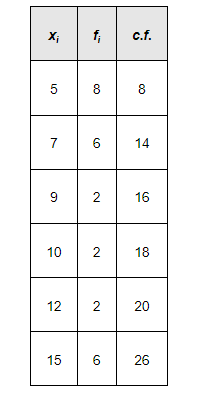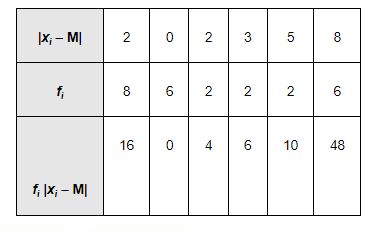# Find the mean deviation about the median for the data.

Question:

Find the mean deviation about the median for the data.Solution:

The given observations are already in ascending order.

Adding a column corresponding to cumulative frequencies of the given data, we obtain the following table.Here, N = 26, which is even.

Median is the mean of $13^{\text {th }}$ and $14^{\text {th }}$ observations. Both of these observations lie in the cumulative frequency 14 , for which the corresponding observation is 7 .

$\therefore$ Median $=\frac{13^{\text {bh }} \text { observation }+14^{\text {ith }} \text { observation }}{2}=\frac{7+7}{2}=7$

The absolute values of the deviations from median, i.e. $\left|x_{i}-M\right|$, are$\sum_{i=1}^{6} f_{i}=26$ and $\sum_{i=1}^{6} f_{i}\left|x_{i}-\mathrm{M}\right|=84$

M.D. $(\mathrm{M})=\frac{1}{\mathrm{~N}} \sum_{i=1}^{6} f_{i}\left|x_{i}-\mathrm{M}\right|=\frac{1}{26} \times 84=3.23$